Ex 12.3

Chapter 12 Class 10 Areas related to Circles (Term 1)
Serial order wise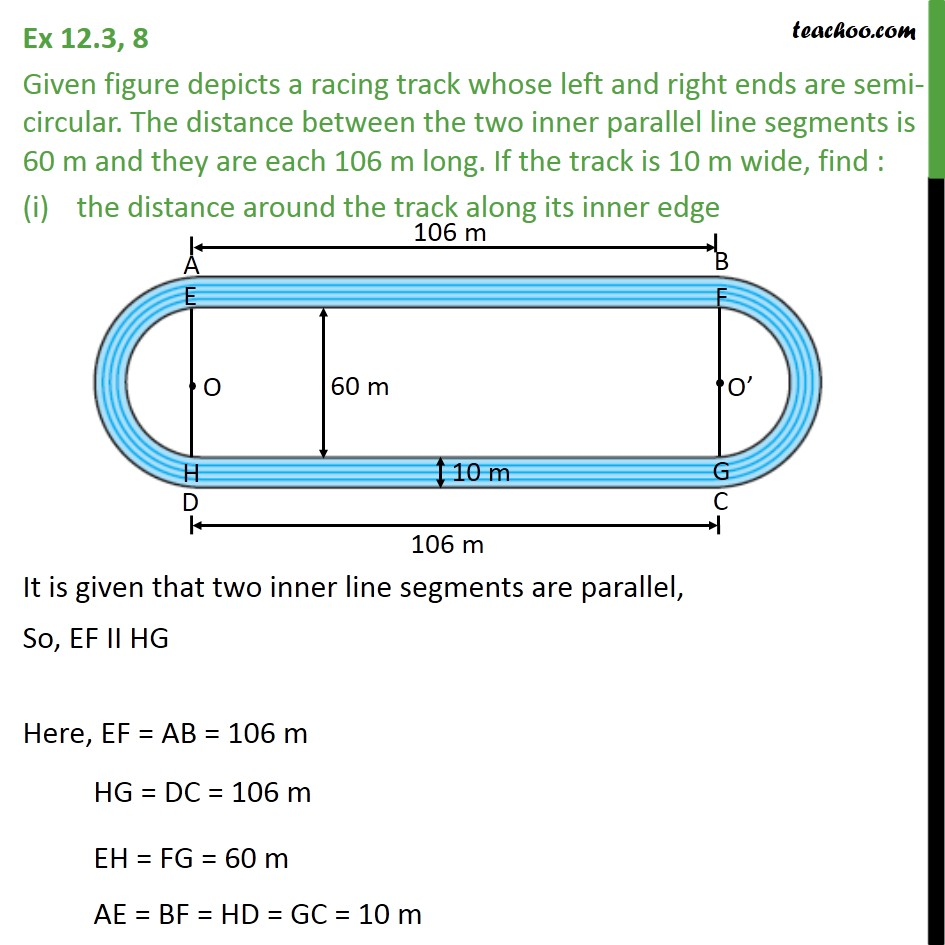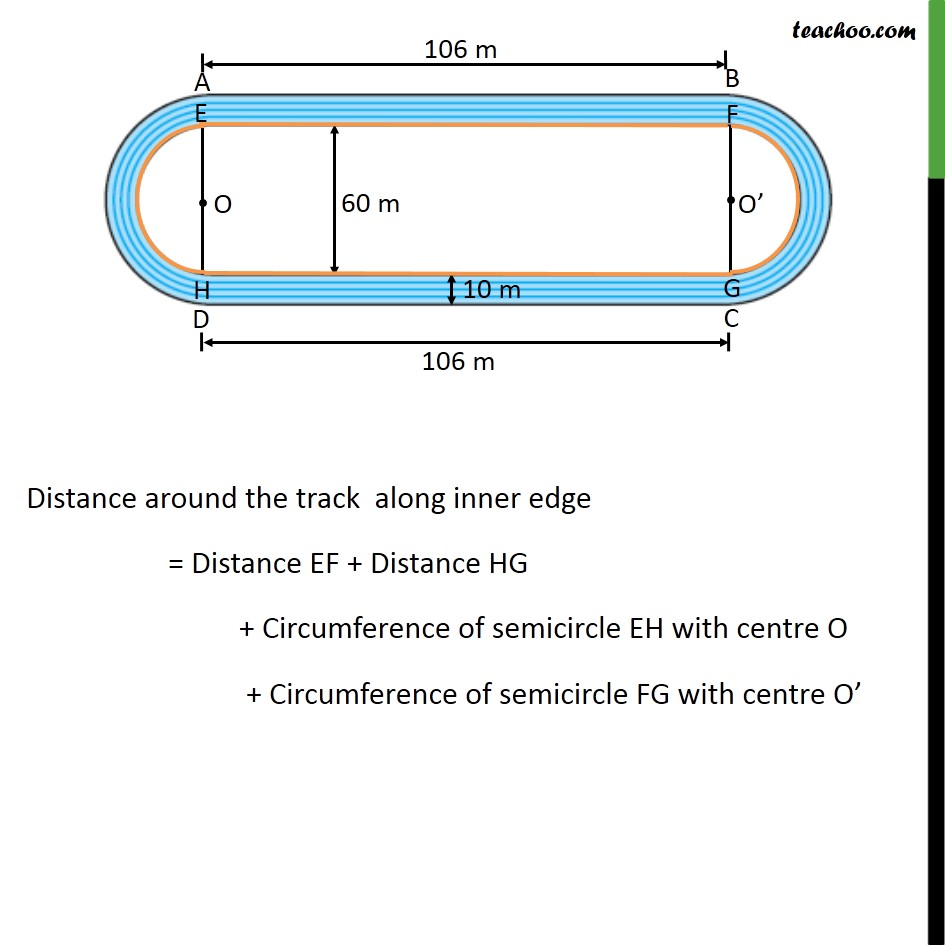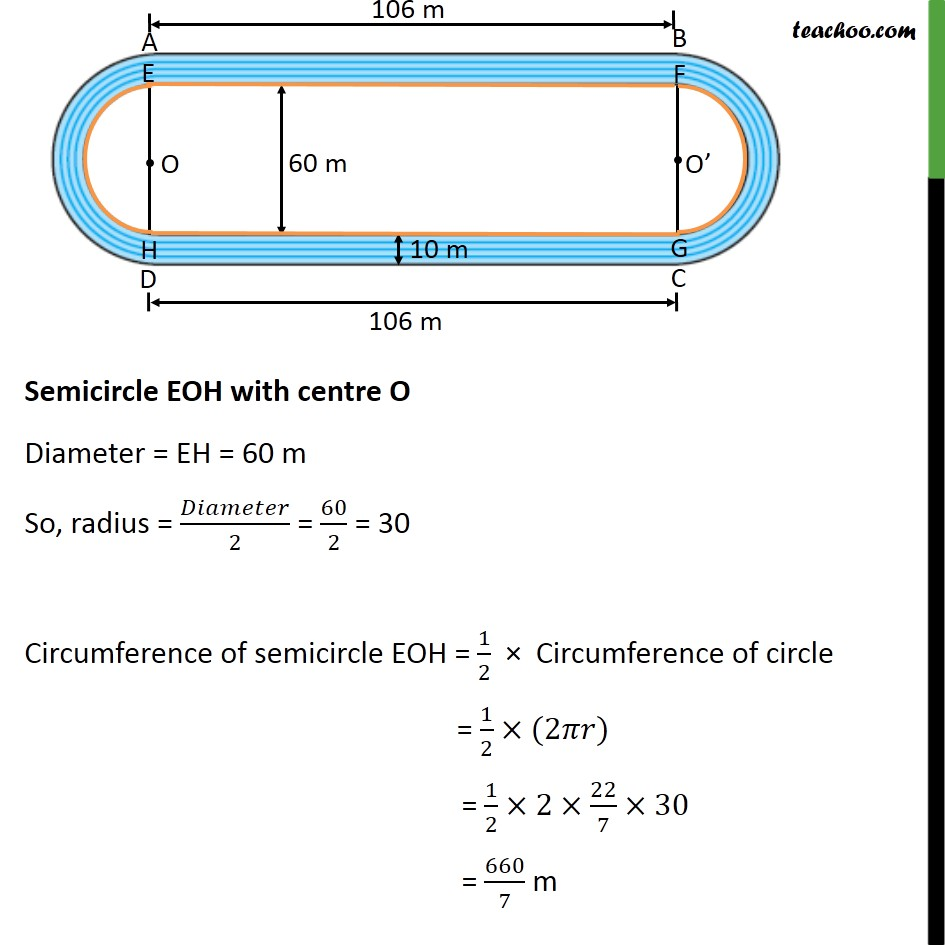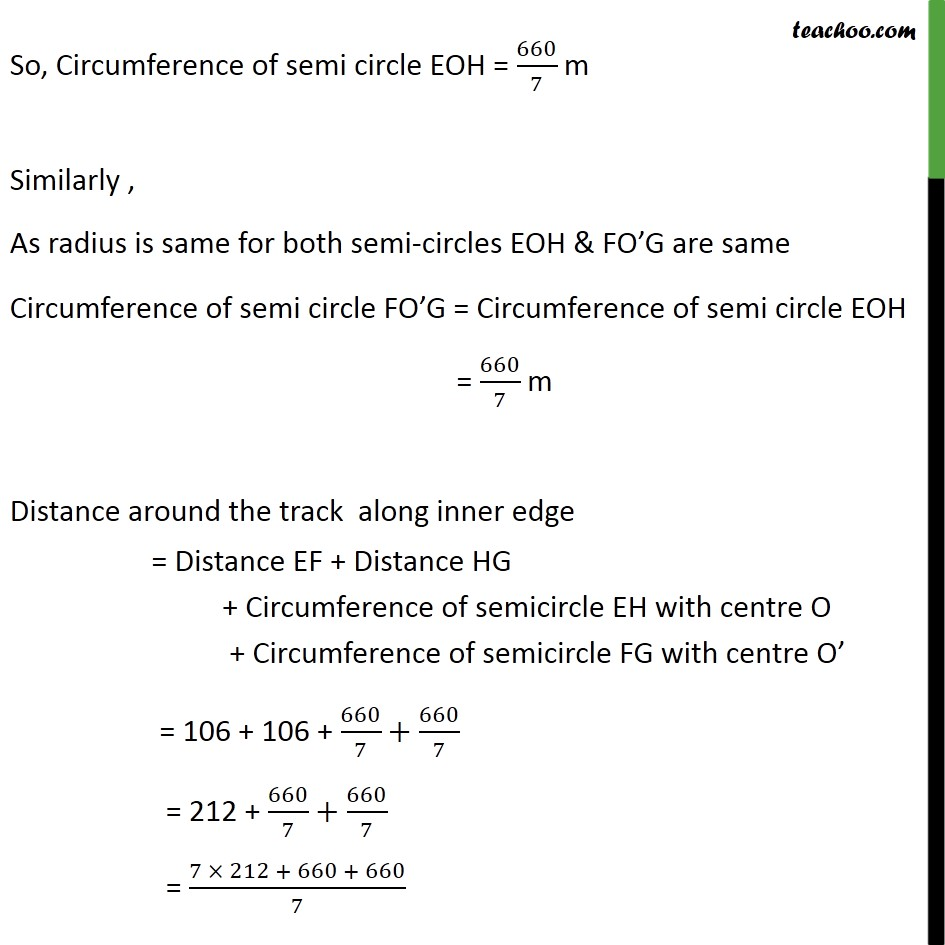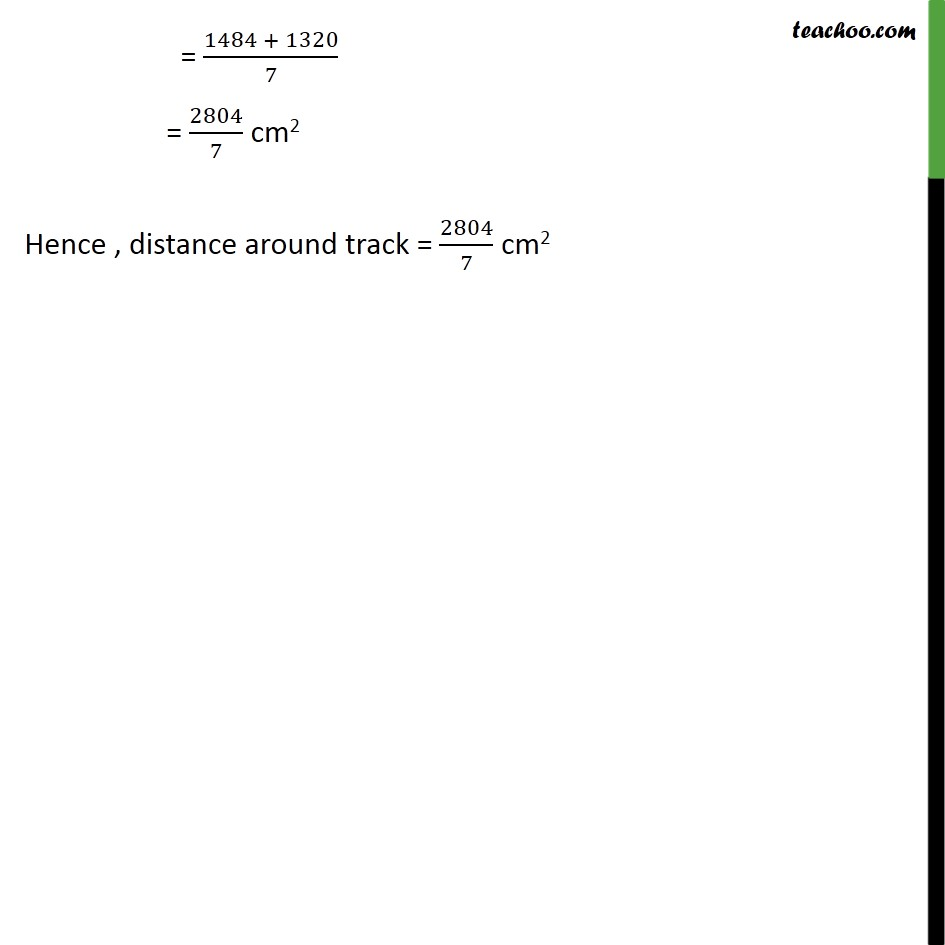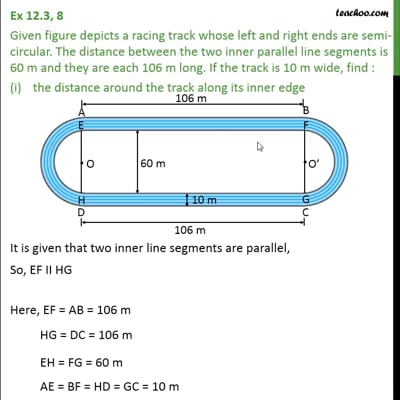This video is only available for Teachoo black users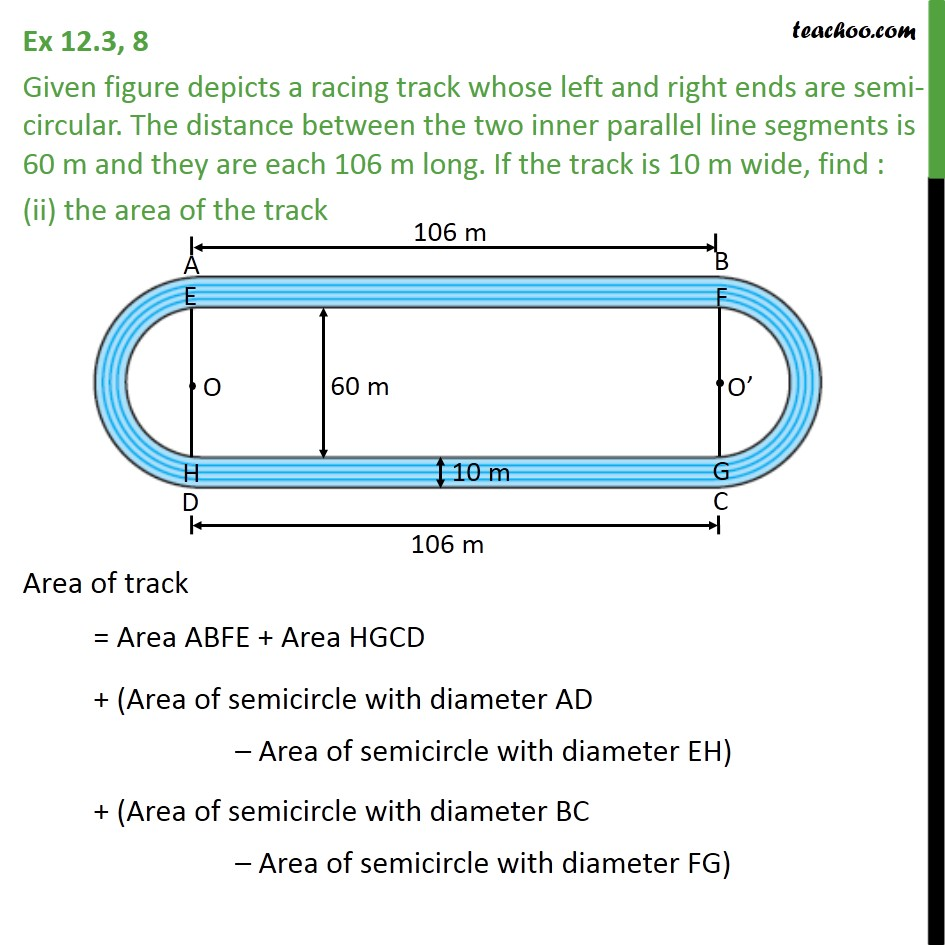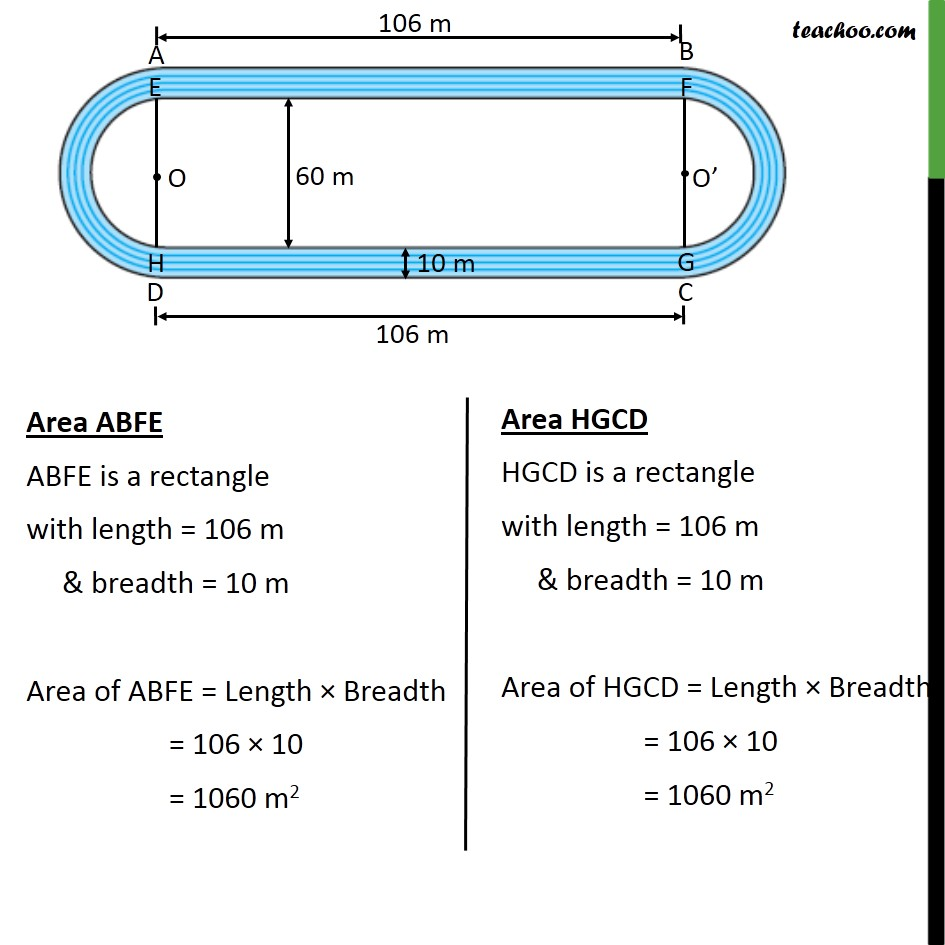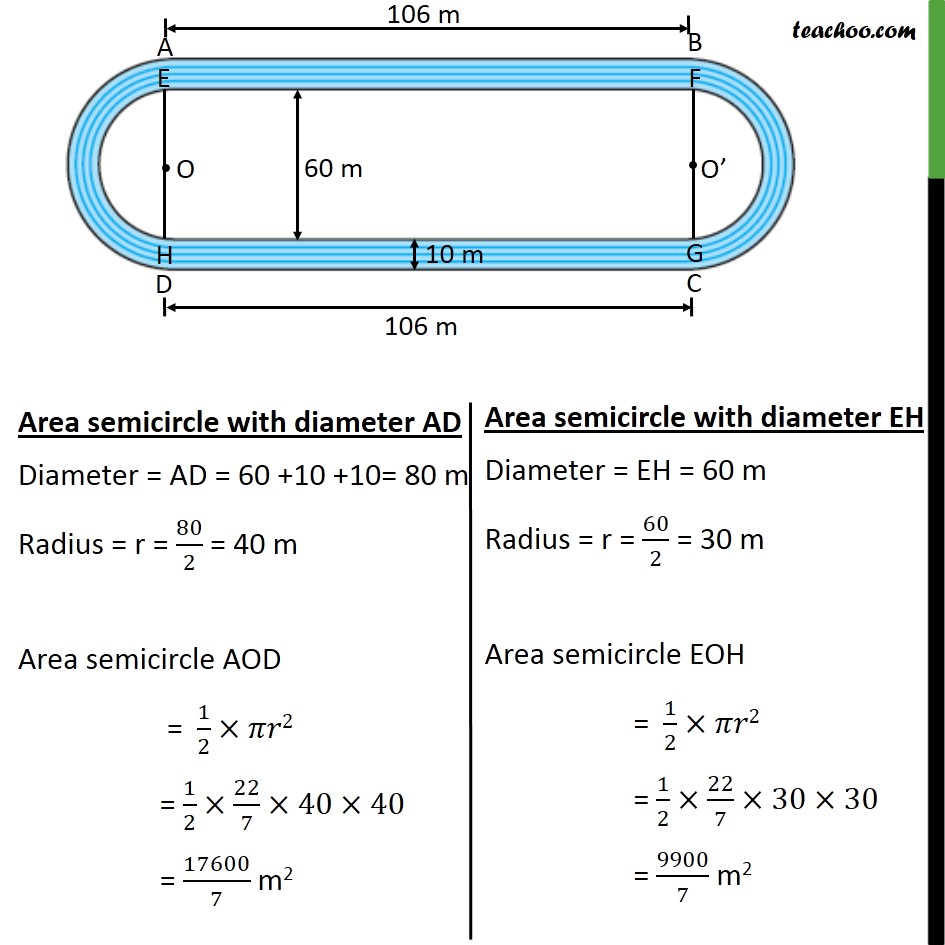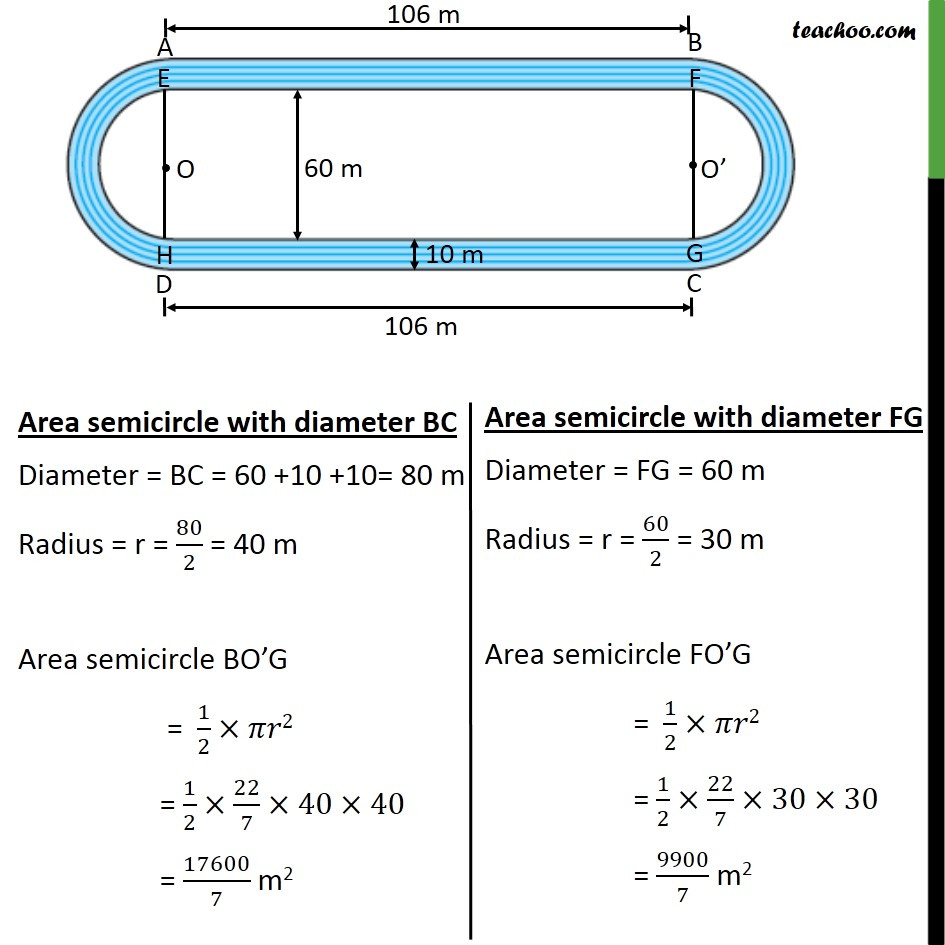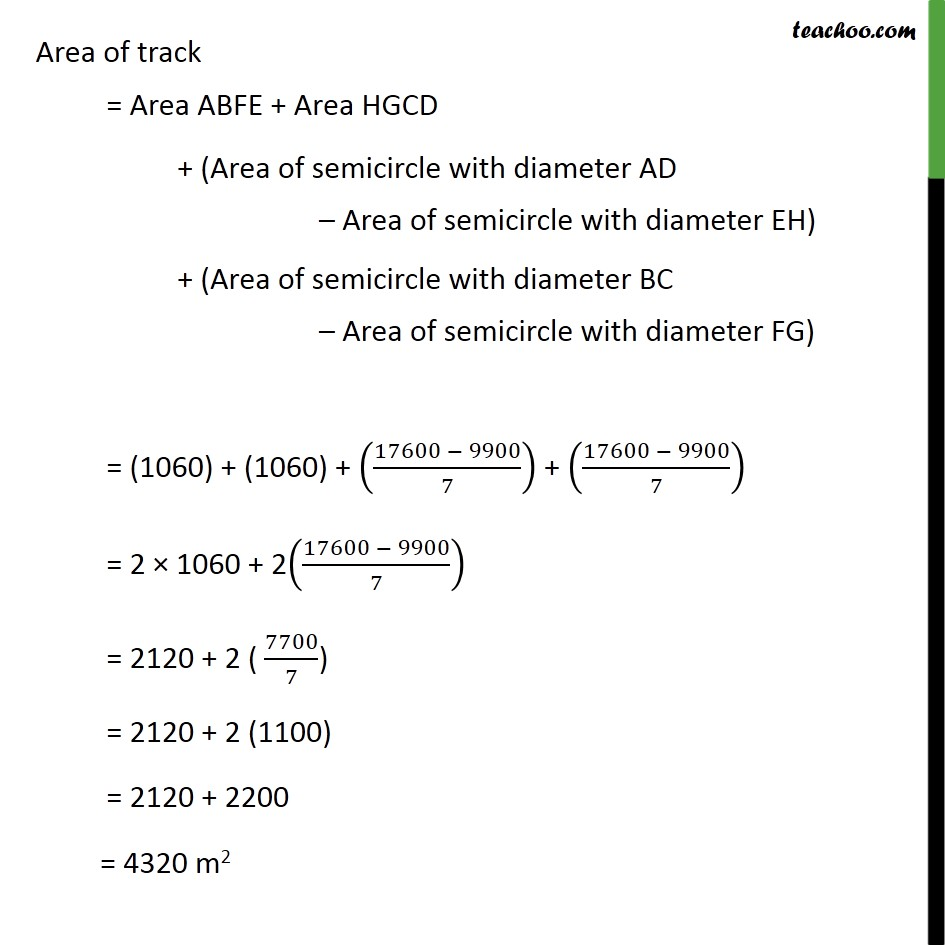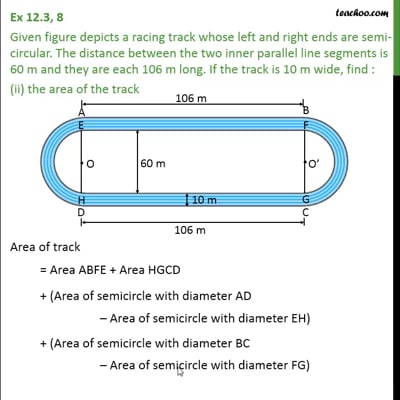This video is only available for Teachoo black users

### Transcript

Ex 12.3, 8 Given figure depicts a racing track whose left and right ends are semi-circular. The distance between the two inner parallel line segments is 60 m and they are each 106 m long. If the track is 10 m wide, find : the distance around the track along its inner edge It is given that two inner line segments are parallel, So, EF II HG Here, EF = AB = 106 m HG = DC = 106 m EH = FG = 60 m AE = BF = HD = GC = 10 m Distance around the track along inner edge = Distance EF + Distance HG + Circumference of semicircle EH with centre O + Circumference of semicircle FG with centre O Semicircle EOH with centre O Diameter = EH = 60 m So, radius = /2 = 60/2 = 30 Circumference of semicircle EOH = 1/2 Circumference of circle = 1/2 (2 ) = 1/2 2 22/7 30 = 660/7 m So, Circumference of semi circle EOH = 660/7 m Similarly , As radius is same for both semi-circles EOH & FO G are same Circumference of semi circle FO G = Circumference of semi circle EOH = 660/7 m Distance around the track along inner edge = Distance EF + Distance HG + Circumference of semicircle EH with centre O + Circumference of semicircle FG with centre O = 106 + 106 + 660/7+660/7 = 212 + 660/7+660/7 = (7 212 + 660 + 660)/7 = (1484 + 1320)/7 = 2804/7 cm2 Hence , distance around track = 2804/7 cm2 Ex 12.3, 8 Given figure depicts a racing track whose left and right ends are semi-circular. The distance between the two inner parallel line segments is 60 m and they are each 106 m long. If the track is 10 m wide, find : (ii) the area of the track Area of track = Area ABFE + Area HGCD + (Area of semicircle with diameter AD Area of semicircle with diameter EH) + (Area of semicircle with diameter BC Area of semicircle with diameter FG) Area ABFE ABFE is a rectangle with length = 106 m & breadth = 10 m Area of ABFE = Length Breadth = 106 10 = 1060 m2 Area of track = Area ABFE + Area HGCD + (Area of semicircle with diameter AD Area of semicircle with diameter EH) + (Area of semicircle with diameter BC Area of semicircle with diameter FG) = (1060) + (1060) + ((17600 9900)/7) + ((17600 9900)/7) = 2 1060 + 2((17600 9900)/7) = 2120 + 2 ( 7700/7) = 2120 + 2 (1100) = 2120 + 2200 = 4320 m2